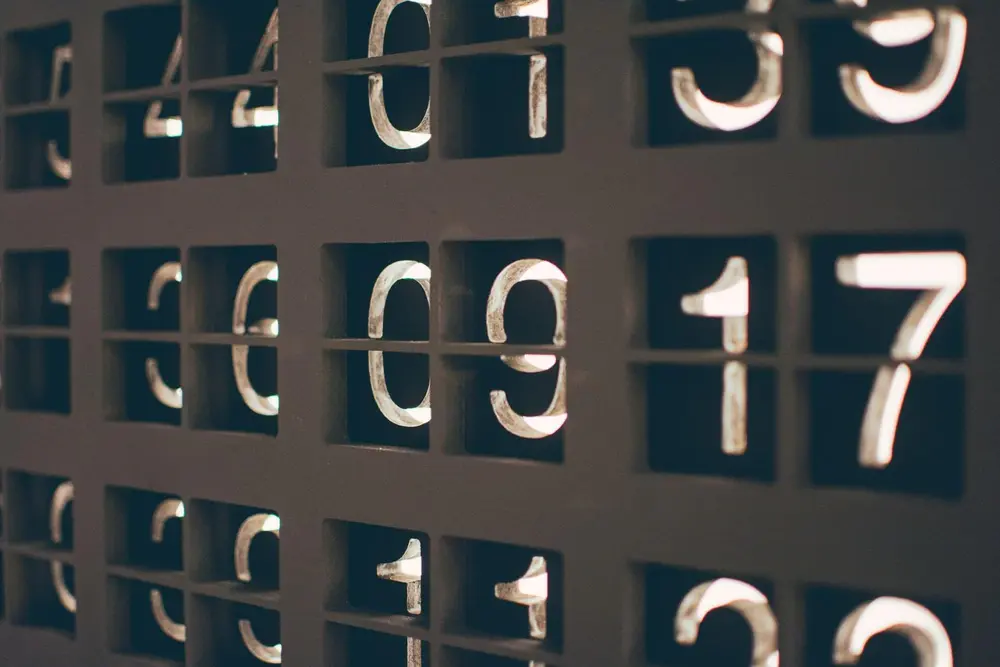# Percentages

• Percentages are used all the time.
• They are used just like fractions.
• Just like fractions, they are used to find part of a value which may be an increase or decrease of the original value.

A percentage is a number out of 100, so:

• 1% is equal to 1/100
• 23% is equal to 23/100
• 50% is equal to 50/100 or 1/2
• When using percentages on a calculator, I find that converting the percentage into a fraction or decimal is more simpler than using the percentage button. Knowing how to convert a percentage would make this process easier.
• Finding percentages of values includes decreases and increases:
• Decreases: A decrease of 10% means 90% of the value ( 100% - 10% = 90% or 0.90 ). To find the answer you work out 0.90 × number.
• Increases: An increase of 10% means your value and an extra 10% added to it. This means it is 110% ( 100% + 10% = 110% or 1.10 ) of the value. To find the answer you work out 1.10 × number.
Number

Number

Number

Number

Number

Number